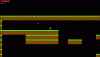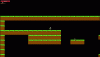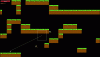# GMS 2.3+Platformer - TileMap Collision Bugs

#### 31Nf4ChZ4H73N

##### Member
Hey I am working on a Platformer Game and I have implemented GM Wolfs tutorial

The first bug I ran into was that my player object which executed the code below in its Step event always got stucked and could not move.I though that this might be an mask related issue so I fixed this by passing mask_index instead of sprite_index as an argument of sprite_get_xoffset. this fixed the problem.

When I used the same code for my enemy object the enemy again got stucked into the tiles.However I fixed this again by passing sprite_index instead of mask_index. The opposite of what I did to the player object. So, this is weird because the enemy sprite also uses a mask which is smaller than the sprite ?

The third bug which occurred was that whenever the object enemy runs into a wall the direction changes and the image_xscale gets the opposite direction. But this makes the enemy fall through the tiles.The Player uses this code for movement and tile collision checks
GML:
``````function move(){
var dist = oGame.dist;
#region Jump
var t1 = tilemap_get_at_pixel(oLevelCreator.backTiles, bbox_left, bbox_bottom + 1) & tile_index_mask;
var t2 = tilemap_get_at_pixel(oLevelCreator.backTiles, bbox_right, bbox_bottom + 1) & tile_index_mask;
if(t1 != 0 || t2 != 0)
{
var jump = ((oInput.pressedLeft>=0)&&(device_mouse_y(oInput.pressedLeft)<oInput.initialLeftTapYViewY-32));
//jump
if(jump&&jumpSwitch){
vspd = (oInput.pressedLeft>=0)*jump*-6;
jumpSwitch = false;
}
if(!jumpSwitch && ((oInput.pressedLeft>=0)&&(device_mouse_y(oInput.pressedLeft)>oInput.initialLeftTapYViewY-32))){
jumpSwitch = true;
}
}
#endregion

var dx = oInput.pressedLeftDir*((oInput.pressedLeft>=0)&&(oInput.pressedLeftDist>dist));
var dy= vspd;
vspd += grav;
#region vertical Movement
y += dy;
if(dy >0){
//sprite_index = sHunterJumpDown;
var t1 = tilemap_get_at_pixel(oLevelCreator.backTiles, bbox_left, bbox_bottom) & tile_index_mask;
var t2 = tilemap_get_at_pixel(oLevelCreator.backTiles, bbox_right, bbox_bottom) & tile_index_mask;
if(t1 != 0 || t2 != 0)
{

y = ((bbox_bottom & ~63)-1) - sprite_bbox_bottom;
vspd = 0;
}
}else
{
var t1 = tilemap_get_at_pixel(oLevelCreator.backTiles, bbox_left, bbox_top) & tile_index_mask;
var t2 = tilemap_get_at_pixel(oLevelCreator.backTiles, bbox_right, bbox_top) & tile_index_mask;
if(t1 != 0 || t2 != 0)
{
//sprite_index = sHunterJumpUp;
y = ((bbox_top +64) & ~63) - sprite_bbox_top;
vspd = 0;
}
}
#endregion
#region horizontal Movement
x += dx*spd;
if(dx!=0)
{
image_xscale = dx;
sprite_index = sHunterRun;
}else
{
sprite_index = sHunterIdle;
}
if(dx >0){
var t1 = tilemap_get_at_pixel(oLevelCreator.backTiles, bbox_right, bbox_top) & tile_index_mask;
var t2 = tilemap_get_at_pixel(oLevelCreator.backTiles, bbox_right, bbox_bottom) & tile_index_mask;
if(t1 != 0 || t2 != 0)
{
x = ((bbox_right & ~63)-1) - sprite_bbox_right;
}
}else
{
var t1 = tilemap_get_at_pixel(oLevelCreator.backTiles, bbox_left, bbox_top) & tile_index_mask;
var t2 = tilemap_get_at_pixel(oLevelCreator.backTiles, bbox_left, bbox_bottom) & tile_index_mask;
if(t1 != 0 || t2 != 0)
{
x = ((bbox_left +64) & ~63) - sprite_bbox_left;
}
}
#endregion
}``````
The Enemy uses this code for movement and tile collision checks
GML:
``````// Script assets have changed for v2.3.0 see
function enemyActive(){
sprite_bbox_left = sprite_get_bbox_left(sprite_index)- sprite_get_xoffset(sprite_index);
sprite_bbox_right = sprite_get_bbox_right(sprite_index)- sprite_get_xoffset(sprite_index);
sprite_bbox_bottom = sprite_get_bbox_bottom(sprite_index)- sprite_get_yoffset(sprite_index);
sprite_bbox_top = sprite_get_bbox_top(sprite_index)- sprite_get_yoffset(sprite_index);
var t1 = tilemap_get_at_pixel(oLevelCreator.backTiles, bbox_left, bbox_bottom + 1) & tile_index_mask;
var t2 = tilemap_get_at_pixel(oLevelCreator.backTiles, bbox_right, bbox_bottom + 1) & tile_index_mask;
if(t1 != 0 || t2 != 0)
{
vspd = 0;
}

var dx = dir;
var dy= vspd;
vspd += grav;

#region vertical Movement
y += dy;
if(dy >0){
//sprite_index = sHunterJumpDown;
var t1 = tilemap_get_at_pixel(oLevelCreator.backTiles, bbox_left, bbox_bottom) & tile_index_mask;
var t2 = tilemap_get_at_pixel(oLevelCreator.backTiles, bbox_right, bbox_bottom) & tile_index_mask;
if(t1 != 0 || t2 != 0)
{
y = ((bbox_bottom & ~63)-1) - sprite_bbox_bottom;
vspd = 0;
}
}else
{
var t1 = tilemap_get_at_pixel(oLevelCreator.backTiles, bbox_left, bbox_top) & tile_index_mask;
var t2 = tilemap_get_at_pixel(oLevelCreator.backTiles, bbox_right, bbox_top) & tile_index_mask;
if(t1 != 0 || t2 != 0)
{
//sprite_index = sHunterJumpUp;
y = ((bbox_top +64) & ~63) - sprite_bbox_top;
vspd = 0;
}
}
#endregion

#region horizontal Movement

x += dx*spd;

if(dx >0){
var t1 = tilemap_get_at_pixel(oLevelCreator.backTiles, bbox_right, bbox_top) & tile_index_mask;
var t2 = tilemap_get_at_pixel(oLevelCreator.backTiles, bbox_right, bbox_bottom) & tile_index_mask;
if(t1 != 0 || t2 != 0)
{
x = ((bbox_right & ~63)-1) - sprite_bbox_right;
dir = dir*-1;

}
}else
{
var t1 = tilemap_get_at_pixel(oLevelCreator.backTiles, bbox_left, bbox_top) & tile_index_mask;
var t2 = tilemap_get_at_pixel(oLevelCreator.backTiles, bbox_left, bbox_bottom) & tile_index_mask;
if(t1 != 0 || t2 != 0)
{
x = ((bbox_left +64) & ~63) - sprite_bbox_left;
dir = dir*-1;

}
}
image_xscale = dir;
#endregion//*/
}``````
So what do I do wrong at all? why does this code not work properly and has to be adjusted for each object?

#### Fanatrick

##### Member
You should not use image_xscale to represent the direction of your actors, flipping it to negative will also affect whichever collision masks you have in place - and I'm guessing since they're not centered your actors end up inside other geometry.

#### 31Nf4ChZ4H73N

##### Member
You should not use image_xscale to represent the direction of your actors, flipping it to negative will also affect whichever collision masks you have in place - and I'm guessing since they're not centered your actors end up inside other geometry.
Actually all the sprites in use are centered. However, what should I change then? just use a flipped sprite?
GML:
``image_xscale = dir``
use something like
GML:
``````if(dir >0){
sprite_index = sprite_enemy_right;
}else{
sprite_index = sprite_enemy_left;
}``````

#### Fanatrick

##### Member
Actually all the sprites in use are centered. However, what should I change then? just use a flipped sprite?
GML:
``image_xscale = dir``
use something like
GML:
``````if(dir >0){
sprite_index = sprite_enemy_right;
}else{
sprite_index = sprite_enemy_left;
}``````
No, best case scenario you would use a custom variable that does not affect the collision mask. I see you're using `dir` already, might just draw these actors with `draw_sprite_ext()`, substitute `image_xscale` for `dir` and leave `image_xscale` alone.

#### Pixel-Team

##### Master of Pixel-Fu
Your tile checks are only testing collisions that are 1 pixel outside your bbox edges, but then you are moving your characters distances greater than 1 pixel, causing them to move into tiles when they shouldn't be able to.

What you're doing is seeing if it's okay to move 1 pixel, and then moving 10 if that proves true.

When you check for a tile collision using the bbox edge, instead of adding 1 pixel, add your object's speed. If you're checking the tile above the object, check his bbox top edge, plus his vspd. You want to test where the object WILL BE, not where he is CURRENTLY.

#### TheouAegis

##### Member
Your tile checks are only testing collisions that are 1 pixel outside your bbox edges, but then you are moving your characters distances greater than 1 pixel, causing them to move into tiles when they shouldn't be able to.
What? His code is post-operative, not pre-operative. He is moving first and then snapping out of collisions after moving. Speed doesn't matter.

Don't use the sprite_get functions. Just use
`bbox_left-image_xoffset`
for example.

#### 31Nf4ChZ4H73N

##### Member
Don't use the sprite_get functions. Just use
`bbox_left-image_xoffset`
for example.
there is no inbuilt `image_xoffset` function?

#### 31Nf4ChZ4H73N

##### Member
ok but there is a `sprite_xoffset` function. I will try this one

#### 31Nf4ChZ4H73N

##### Member
What? His code is post-operative, not pre-operative. He is moving first and then snapping out of collisions after moving. Speed doesn't matter.

Don't use the sprite_get functions. Just use
`bbox_left-image_xoffset`
for example.
Ok I tried ` bbox_left - sprite_xoffset ` instead of `sprite_get_bbox_left(sprite_index)- sprite_get_xoffset(sprite_index);` but this just teleports the object to the left corner of the room.

You should not use image_xscale to represent the direction of your actors, flipping it to negative will also affect whichever collision masks you have in place - and I'm guessing since they're not centered your actors end up inside other geometry.
I just checked the sprites again and you were right there were not centered. I fixed this and now the bug that the enemy falls through the tiles is fixed.

however, I still do not understand why I have to use sprite x offset from the `mask_index` for the player object and why for the enemy object only the sprite xxoffset from the `sprite_index` works. Any idea? they both use mask which are smaller than their sprite.